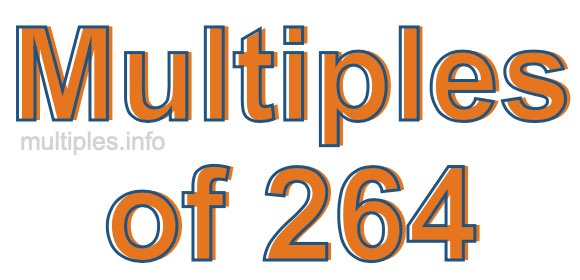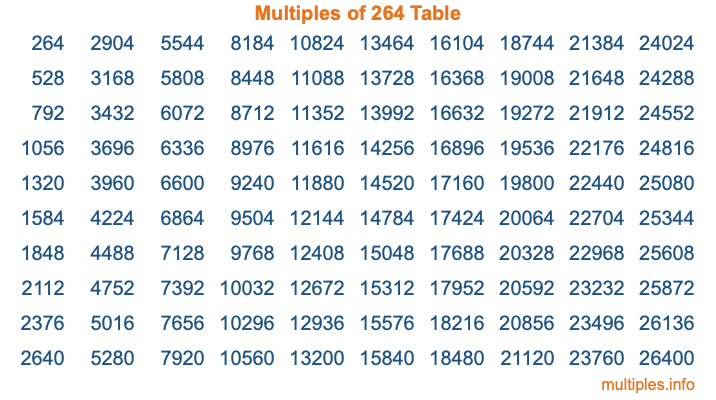Multiples of 264Welcome to the Multiples of 264 page. Here we will first teach you everything you will ever need to know about the multiples of 264, and then give you a study guide summary of everything we taught you to make sure you remember it all. Use this page to look up facts and learn information about the multiples of 264. This page will make you a multiples of two hundred sixty-four expert!

Definition of Multiples of 264
Multiples of 264 are all the numbers that when divided by 264 equal an integer. Each of the multiples of 264 are called a multiple. A multiple of 264 is created by multiplying 264 by an integer.

Therefore, to create a list of multiples of 264, you start with 1 multiplied by 264, then 2 multiplied by 264, then 3 multiplied by 264, and so on for as long as you want. Thus, the list of the first five multiples of 264 is 264, 528, 792, 1056, and 1320. To see a larger list of multiples of 264, see the printable image of Multiples of 264 further down on this page. We also have a category where you can choose any nth multiple of 264.

Multiples of 264 Checker
The Multiples of 264 Checker below checks to see if any number of your choice is a multiple of 264. In other words, it checks to see if there is any number (integer) that when multiplied by 264 will equal your number. To do that, we divide your number by 264. If the the quotient is an integer, then your number is a multiple of 264.

Is  a multiple of 264?

Least Common Multiple of 264 and ...
A Least Common Multiple (LCM) is the lowest multiple that two or more numbers have in common. This is also called the smallest common multiple or lowest common multiple and is useful to know when you are adding our subtracting fractions. Enter one or more numbers below (264 is already entered) to find the LCM.

Check out our LCM Calculator if you need more details about the Least Common Multiple or if you need the LCM for different numbers for adding and subtraction fractions.

nth Multiple of 264
As we stated above, 264 is the first multiple of 264, 528 is the second multiple of 264, 792 is the third multiple of 264, and so on. Enter a number below to find the nth multiple of 264.

th multiple of 264

Multiples of 264 vs Factors of 264
264 is a multiple of 264 and a factor of 264, but that is where the similarities end. All postive multiples of 264 are 264 or greater than 264. All positive factors of 264 are 264 or less than 264.

Below is the beginning list of multiples of 264 and the factors of 264 so you can compare:

Multiples of 264: 264, 528, 792, 1056, 1320, etc.

Factors of 264: 1, 2, 3, 4, 6, 8, 11, 12, 22, 24, 33, 44, 66, 88, 132, 264

As you can see, the multiples of 264 are all the numbers that you can divide by 264 to get a whole number. The factors of 264, on the other hand, are all the whole numbers that you can multiply by another whole number to get 264.

It's also interesting to note that if a number (x) is a factor of 264, then 264 will also be a multiple of that number (x).

Multiples of 264 vs Divisors of 264
The divisors of 264 are all the integers that 264 can be divided by evenly. Below is a list of the divisors of 264.

Divisors of 264: 1, 2, 3, 4, 6, 8, 11, 12, 22, 24, 33, 44, 66, 88, 132, 264

The interesting thing to note here is that if you take any multiple of 264 and divide it by a divisor of 264, you will see that the quotient is an integer.

Multiples of 264 Table
Below is an image of the first 100 multiples of 264 in a table. The table is in chronological order, column by column. The first column has the first ten multiples of 264, the second column has the next ten multiples of 264, and so on.The Multiples of 264 Table is also referred to as the 264 Times Table or Times Table of 264. You are welcome to print out our table for your studies.

Negative Multiples of 264
Although not often discussed or needed in math, it is worth mentioning that you can make a list of negative multiples of 264 by multiplying 264 by -1, then by -2, then by -3, and so on, to get the following list of negative multiples of 264:

-264, -528, -792, -1056, -1320, etc.

Multiples of 264 Summary
Below is a summary of important Multiples of 264 facts that we have discussed on this page. To retain the knowledge on this page, we recommend that you read through the summary and explain to yourself or a study partner why they hold true.

There are an infinite number of multiples of 264.

A multiple of 264 divided by 264 will equal a whole number.

264 divided by a factor of 264 equals a divisor of 264.

The nth multiple of 264 is n times 264.

The largest factor of 264 is equal to the first positive multiple of 264.

264 is a multiple of every factor of 264.

264 is a multiple of 264.

A multiple of 264 divided by a divisor of 264 equals an integer.

264 divided by a divisor of 264 equals a factor of 264.

Any integer times 264 will equal a multiple of 264.

Multiples of a Number
Here you can get the multiples of another number, all with the same attention to detail as we did for multiples of 264 on this page.

Multiples of
Multiples of 265
Did you find our page about multiples of two hundred sixty-four educational? Do you want more knowledge? Check out the multiples of the next number on our list!